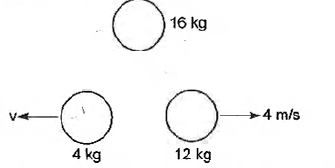Question

# A bomb of mass 16kg at rest, explodes into two pieces of masses 4kg and 12kg. After explosion, the velocity of the 12 kg mass is 4 m/s. What is the velocity of the 4 kg piece?

Open in App
Solution

## Step 1: Given dataThe mass of the bomb is $16kg.$The mass of the first piece is $4kg$.The mass of the second piece is ${m}_{1}=12kg$ and its velocity is ${v}_{2}=4m/s.$ If a bomb of mass $M$ explodes into two pieces of mass ${m}_{1}$ and ${m}_{2}$ with velocities ${v}_{1}$ and ${v}_{2}$,Then from the concept of conservation of linear momentum, we know that the linear momentum of a system of particles remains constant in absence of external force, i.e, $MV={m}_{1}{v}_{1}+{m}_{2}{v}_{2}$, where $V$ is the velocity of the bomb.DiagramStep 2: Find the velocity of the second piece Let, ${m}_{1}$ and ${m}_{2}$ are the mass of the two pieces with velocities ${v}_{1}$ and ${v}_{2}$,From the principle of conservation of linear momentum,$MV={m}_{1}{v}_{1}+{m}_{2}{v}_{2}$$0=+\left(4×{v}_{1}\right)+\left(12×4\right)\phantom{\rule{0ex}{0ex}}or{v}_{1}=-\frac{48}{4}=-12.\phantom{\rule{0ex}{0ex}}or{v}_{1}=-12m/s.$Neglecting the minus sign of velocity, because the two masses are moving in opposite directions from each other.So, the velocity of the first body is $12m/s$.Suggest Corrections0Similar questions